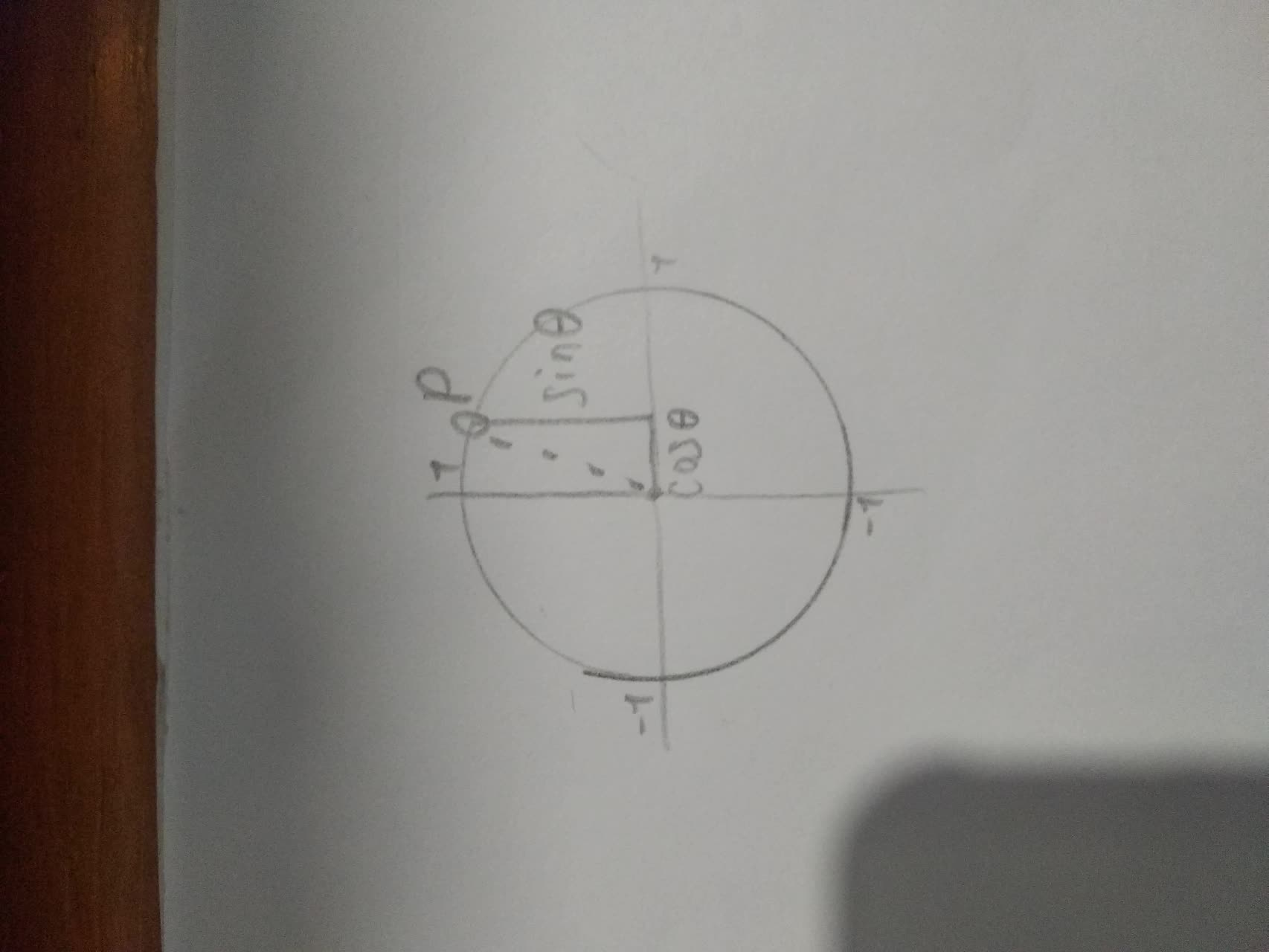# Why are the trigonometric functions sometimes called circular functions?jazzcutie0h 2021-11-17 Answered
Why are the trigonometric functions sometimes called circular functions?
You can still ask an expert for help

• Questions are typically answered in as fast as 30 minutes

Solve your problem for the price of one coffee

• Math expert for every subject
• Pay only if we can solve itmylouscrapza

Trigonometric functions are called circular functions because function values can be expressed in terms of coordinates of a point on a unit circle.
These trignometric functions are called circular functions because radian measures of angles are determined by the lengths of arcs of circles.The sine and cosine function are defined as the coordinates of a point P on a circle of radius 1.
.
Hence, trigonometric functions are called circular functions because function values can be expressed in terms of coordinates of a point on a unit circle.